## Do we need Crossover?

At a certain point of time t the set of genotypes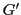in a working population of phenotypes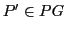(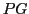as the set of all possible phenotypes) can have - in the worst case - only genes (:= information strings) which are not in the success set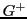, written as. The interesting question is whether we need besides mutation to change the content ofalso the crossover operation.

The 'classical' operators of the Genetic Algorithm Paradigm (GAP) are

1. reproduction (selection) is based on available fitness values indicating the 'goodness' of a working information pattern. Reproduction selects from a population the 'good' ones and multiplies them thereby minimizing the 'bad' ones (cf. Goldberg :10f, Suzuki :656).
2. crossover as an exchange between two information patterns selecting a 'cut point' 'x' by random (with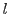representing the length of the information string) and exchanging all elements 'after the cut pointpoint by point (cf. Goldberg :12).
3. mutation as a random choice of some position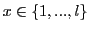where the actual value atwill be replaced by some other value out of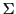(cf. Goldberg :14).

The interesting question is, whether and how it is possible that the working set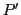can be 'changed' in the 'direction' given by the evaluation function.

The reproduction operation getting some feedback with 'fitness values' can 'improve a given working set, but as long as this set will not change this feedback will stay the same and nothing will happen. A 'bad' working setwill stay as a 'bad' set forever.

The mutation operation can change an information string either in the direction of a 'better' string with regard to the 'unknown' success setor not.

The interesting question here is, what exactly is the role of the crossover operation? Can crossover improve a set of working information strings too or not?

In the section before 5.1 I had stated (without a proof (this was the failure)), that crossover can not improve a working set. As we will see below there are very special circumstances where crossover can improve a working setand this happens at best in close 'cooperation' with mutation.

Let us assume as test set.

Applying crossover in this case would not change anything because with cut-point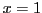all the output of crossover would be already elements of the set.

Asking for those conditions under which crossover can produce some 'new' elements not already contained in the 'old' set -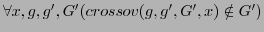- we have to assume a 'canonical' set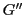where every pair of members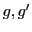is 'different' in the sense that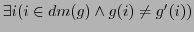(on account of reproduction working sets will usually have many instances of the same pattern).

Assuming a canonical setwe can re-formulate the question asking for those conditions under which the crossover operation can generate a pattern which is not yet an element of the canonical set.

Assuming a test set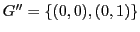shows, that with cut-pointno new element will be generated --.Thus there must be some other special condition under which crossover can produce something 'new'.

Looking to examples like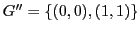,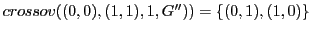,,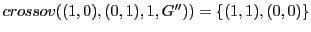that a 'new' pattern can only emerge if values 'before' and 'after' the cut-point are simultaneously exchanged and at all positions in the domain of the patterns the values are different before the exchange. Thus we postulate a kind of asymmetry where we have two patternswith lengthand cut-pointwhere there exists at least one position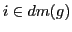'before' the cut-point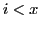and at least one positionwhich is 'after' the cut-point x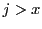and the values at these positions are different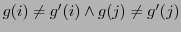. Under this asymmetry-condition we would get new patterns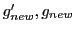with the new elementsand. Argument: Forwe know according to the assumption thatwas specific for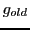it is still specific andis 'new' in combination with. The same holds forrespectively.

But this lets open the question whether the new elements are new with regard to the whole set. For a canonical set we have only postulated that for all pairs there must exists at least 'one' position where they differ. Assume that there would exist one elementwhich would differ withand with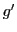in at least one position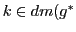.

If this position would be different to, then this difference would be still valid, because the value at this position would not change in. But then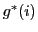could be equal toat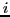. If this would be the case there must exist another position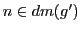wherediffer. This must not necessarily be at position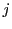. With the assumption so far one cannot exclude that anothercould be equal towhile differing with. This argument applies also to the other sub cases.

From this we have to conclude that the condition for the 'newness' of crossover generated patterns can only be guaranteed if one postulates for (i) local asymmetry and (ii) uniqueness.

We have a local asymmetry between two patternswith lengthand cut-pointif there exists at least one position'before' the cut-pointand at least one positionwhich is 'after' the cut-point xand the values at these positions are different. The distinguishing positions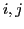are called the asymmetry indexes. Under this asymmetry-condition we would get new patternswith the new elementsand.

We will have uniqueness of two local asymmetric patternsin a working setif this working set is a canonical setand it holds that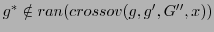.

This definition puts the 'hard work' of revealing the details to some additional proof. But even in this rough format it becomes clear that the usage of crossover is very limited. I will show up that finally crossover makes only sense if a mutation operation has introduced 'enough new patterns' to make crossover applicable.

Gerd Doeben-Henisch 2013-01-14